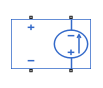# PVCCS

Polynomial voltage-controlled current source

• Library:
• Simscape / Electrical / Additional Components / SPICE Sources

•## Description

The PVCCS (Polynomial Voltage-Controlled Current Source) block represents a current source whose output current value is a polynomial function of the voltage across the input ports. The following equations describe the current through the source as a function of time:

• If you specify an n-element vector of polynomial coefficients for the Polynomial coefficients parameter:

`${I}_{out}=p\left(0\right)+p\left(1\right)*{V}_{in}+...+p\left(n-1\right)*{V}_{in}^{n-1}+p\left(n\right)*{V}_{in}^{n}$`

• If you specify a scalar coefficient for the Polynomial coefficients parameter:

`${I}_{out}=p*{V}_{in}$`

where:

• Vin is the voltage across the input ports.

• p is the Polynomial coefficients parameter value.

The block uses a small conductance internally to prevent numerical simulation issues. The conductance connects the output ports of the device and has a conductance GMIN:

• By default, GMIN matches the GMIN parameter of the Environment Parameters block, whose default value is `1e–12`.

• To change GMIN, add an Environment Parameters to your model and set the GMIN parameter to the desired value.

## Ports

Refer to the figure for port locations.### Conserving

expand all

Electrical conserving port associated with the PVCCS positive voltage of the controlling input.

Electrical conserving port associated with the PVCCS negative voltage of the controlling input.

Electrical conserving port associated with the PVCCS positive output voltage.

Electrical conserving port associated with the PVCCS negative output voltage.

## Parameters

expand all

The polynomial coefficients that relate the input voltage to the output current, as described in the preceding section.

Specify if the current source includes a parallel conductance. The value of the parallel conductance, GMIN, depends on whether your model contains an Environment Parameters block.

This table shows the possible values of the parallel conductance, GMIN:

Values of parallel conductance, GMIN

Include parallel conductance, GMIN
`Yes``No`
Model contains Environment Parameters blockModel does not contain Environment Parameters blockParallel conductance, GMIN, is not included and it does not affect the current source.
GMIN is equal to the value of the GMIN parameter in the Environment Parameters block.GMIN = `1e-12` `Ohm`

## Version History

Introduced in R2008a